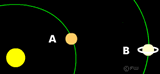## Kepler's Laws Calculations

##### Question 1

Jupiter takes 11.8 years to orbit the Sun. It is 5.2 AU from the Sun. Show how these are related.

T = 11.8 x 11.8 = 140
R = 5.2 x 5.2 x 5.2 = 140

##### Question 2

Saturn orbits the Sun in 29.45 years. What is its orbital radius?

T2 = 29.45 x 29.45 = 867
Cube root of 867 = 9.53. r = 9.53 AU

##### Question 3

Study the image below.
a) Planet A is 3 AU from the Sun. Using Kepler's Third Law find out the time it takes to orbit the Sun.
b) Planet B takes 10 years to orbit the Sun, Find out the distance from the Sun.A)
33 = 27
27 square root = 5.196 AU

B)
102 = 100
100 cube root = 4.642 AU

###### Summary
1. Planets move in elliptical orbits around the Sun.
2. Planets move faster the nearer they are to the Sun.
3. There is a relationship between the distance of a planet and the time it takes to orbit the Sun.

Note: Incidentally some astronomers, textbooks and websites uses "P" for Period rather than "T" for Time. Don't worry if you see this being used elsewhere